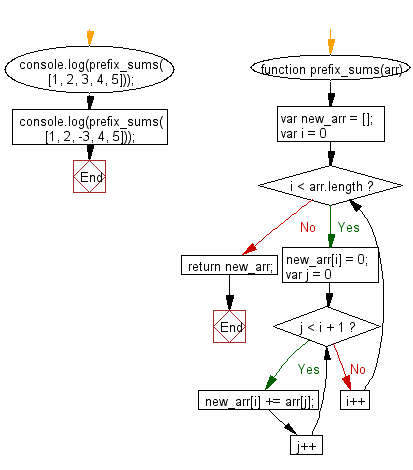# JavaScript: Create an array of prefix sums of the given array

## JavaScript Basic: Exercise-131 with Solution

Write a JavaScript program to create an array of prefix sums of the given array.

In computer science, the prefix sum, cumulative sum, inclusive scan, or simply scan of a sequence of numbers x0, x1, x2, ... is a second sequence of numbers y0, y1, y2, ..., the sums of prefixes of the input sequence:
y0 = x0
y1 = x0 + x1
y2 = x0 + x1+ x2
...

Sample Solution:

HTML Code:

``````<!DOCTYPE html>
<html>
<meta charset="utf-8">
<meta name="viewport" content="width=device-width">
<title>Create an array of prefix sums of the given array</title>
<body>

</body>
</html>
```
```

JavaScript Code:

``````function prefix_sums(arr) {
var new_arr = [];
for (var i = 0; i < arr.length; i++) {
new_arr[i] = 0;
for (var j = 0; j < i + 1; j++) {
new_arr[i] += arr[j];
}
}
return new_arr;
}

console.log(prefix_sums([1, 2, 3, 4, 5]));

console.log(prefix_sums([1, 2, -3, 4, 5]));
``````

Sample Output:

```[1,3,6,10,15]
[1,3,0,4,9]
```

Flowchart:ES6 Version:

``````function prefix_sums(arr) {
const new_arr = [];
for (let i = 0; i < arr.length; i++) {
new_arr[i] = 0;
for (let j = 0; j < i + 1; j++) {
new_arr[i] += arr[j];
}
}
return new_arr;
}

console.log(prefix_sums([1, 2, 3, 4, 5]));

console.log(prefix_sums([1, 2, -3, 4, 5]));
``````

Live Demo:

See the Pen javascript-basic-exercise-131 by w3resource (@w3resource) on CodePen.

Improve this sample solution and post your code through Disqus

What is the difficulty level of this exercise?

Test your Programming skills with w3resource's quiz.

﻿

## JavaScript: Tips of the Day

Checks if a string is an anagram of another string (case-insensitive, ignores spaces, punctuation and special characters)

Example:

```const isAnagram = (str1, str2) => {
const normalize = str =>
str
.toLowerCase()
.replace(/[^a-z0-9]/gi, '')
.split('')
.sort()
.join('');
return normalize(str1) === normalize(str2);
};
console.log(isAnagram('iceman', 'cinema')); // true
```

Output:

```true
```